Maths Challenge 4# Maths Challenge 4

## 1: Planetary Racing Speed

The Earth is 93 million miles from the sun.
This distance is called an Astronomical Unit (AU).
Below is a table of the planets in our solar system, their distance from the sun and the length of their years in Earth time.

Planet Distance from the Sun (AU) Year length in Earth time
Mercury 0.39 88 days
Venus 0.723 225 days
Earth 1 365 days
Mars 1.524 687 days
Jupiter 5.203 11.86 years
Saturn 9.539 29.5 years
Uranus 19.18 84 years
Neptune 30.06 165 years

Using this information, whilst assuming all orbits are circular, which planet is moving the fastest and which is the slowest?

speed = distance ÷ time.
orbit/distance = circumference = 2πr (where r = distance from the sun)

Using π = 3.14 and year = 365 days
speed (in AU per day) = circumference ÷ time in days
Mercury is the fastest planet travelling at 0.28AU a day.

## 2: Number Crossword Basic Operations

To make this more challenging, do not use a calculator.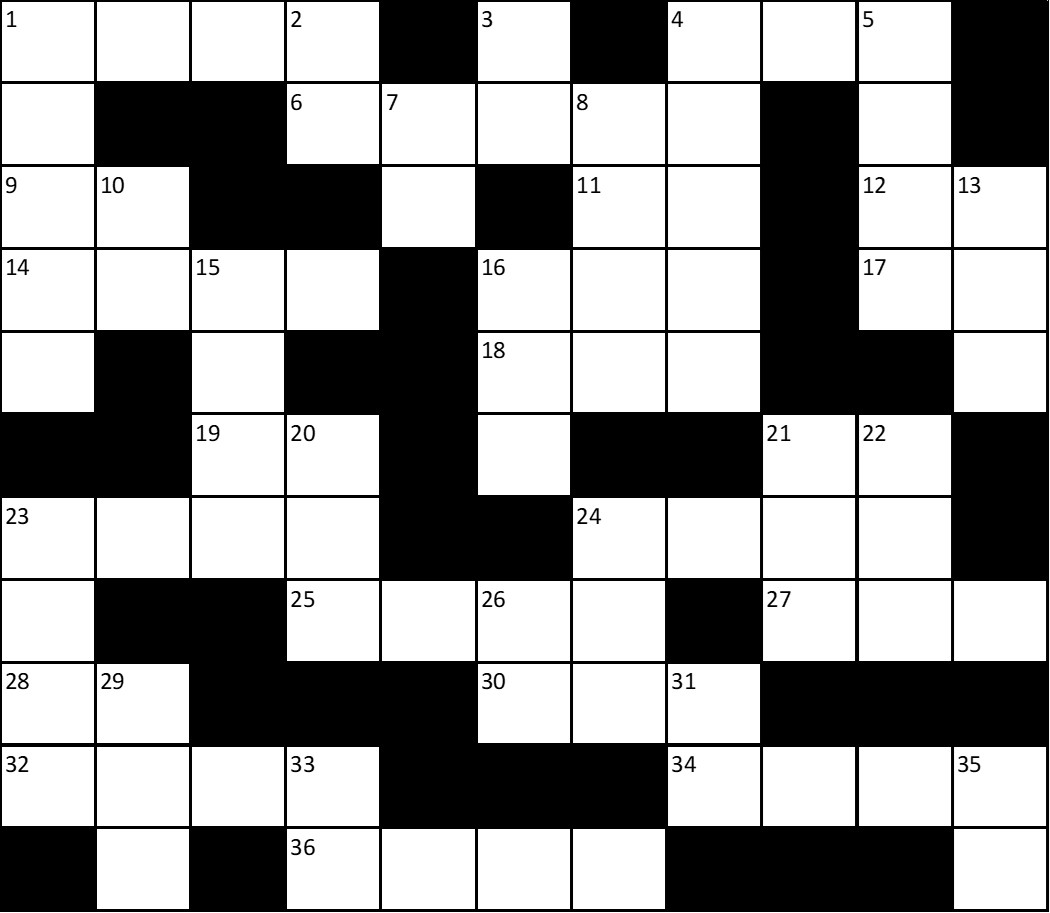Excel version of the crossword grid for screen readers

ACROSS DOWN
1. 1790 + 2686 1. 40433 x 1
4. 8880 ÷ 10 2. 94 - 29
6. 318 x 161 3. 14 - 3
9. 480 ÷ 10 4. 359 x 247
11. 1122 ÷ 17 5. 94 x 86
12. 415 ÷ 5 7. 16 - 4
14. 70 x 43 8. 3564 + 6061
16. 727 x 1 10. 32 + 48
17. 280 ÷ 7 13. 2464 ÷ 8
18. 936 - 283 15. 390 + 704
19. 36 + 63 16. 1087 - 319
21. 6 +12 20. 83 x 11
23. 5741 x 1 21. 281 - 82
24. 3598 -999 22. 356 + 535
25. 5360 - 1576 23. 57780 ÷ 10
27. 7304 ÷ 8 24. 344 - 104
28. 1584 ÷ 22 26. 820 ÷ 10
30. 19 x 11 29. 71 + 135
32. 11264 - 3218 31. 1683 ÷ 17
34. 3378 + 5744 33. 23 x 3
36. 3533 + 6361 35. 29 x 1

Pdf Version Available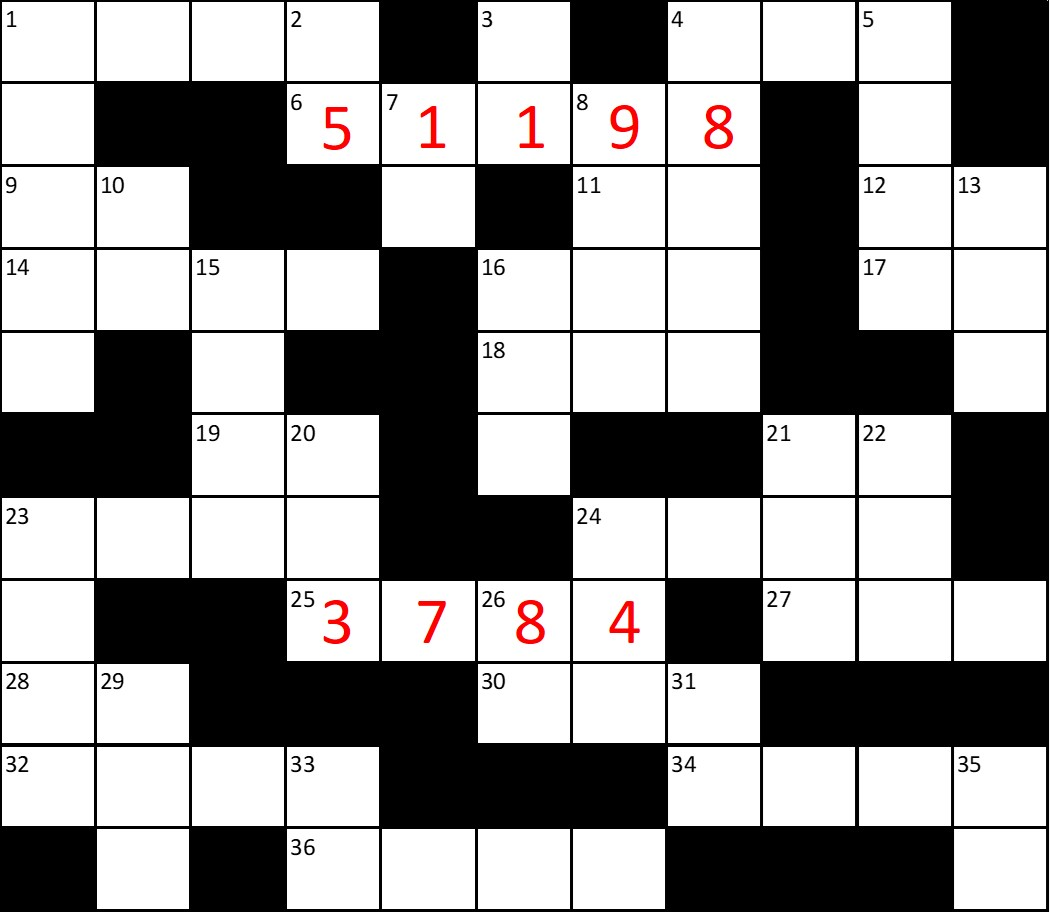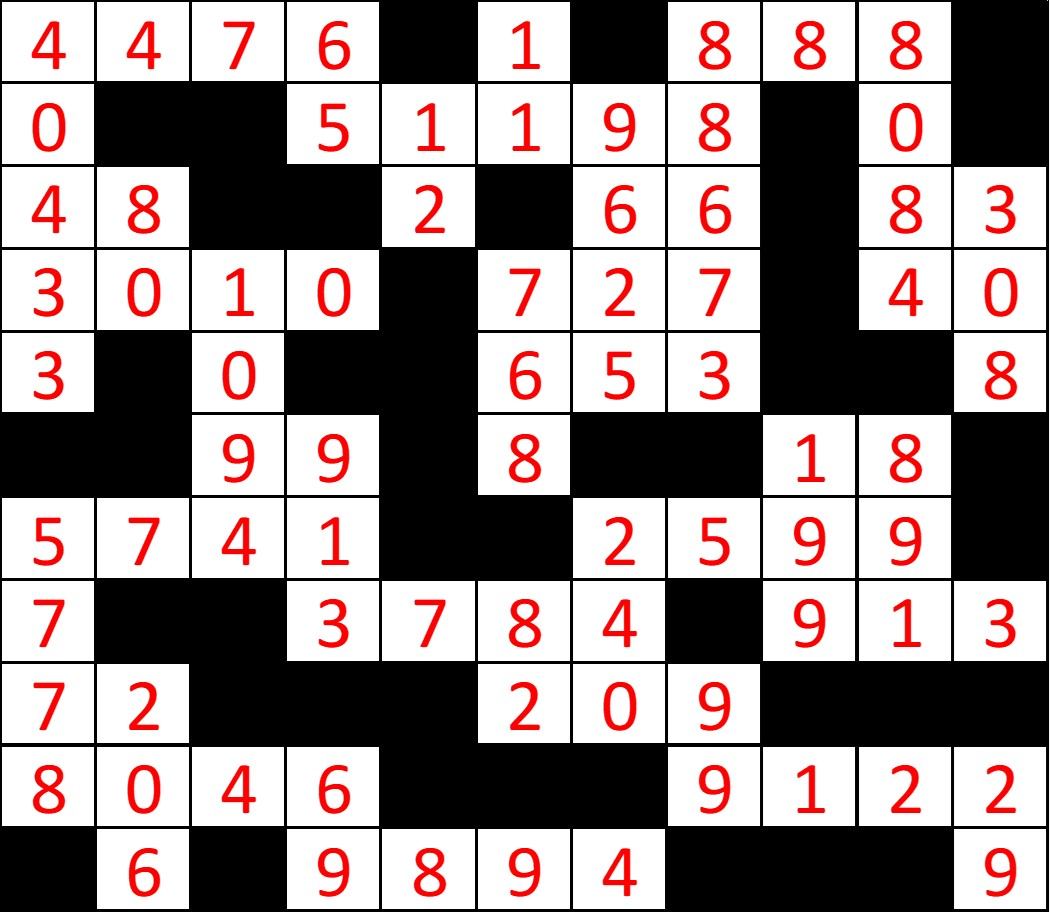Excel version of the completed crossword for screen readers

## 3: Fractions of FractionsFractions

What is the value of one-half of two-thirds of three-quarters of four-fifths of five-sixths of six-sevenths of seven-eighths of eight-ninths of nine-tenths of one thousand?

The trick is to work backwards through this problem, so start with 9/10 of 1000.

nine-tenths of 1000 = 900
eight-ninths of 900 = 800
seven-eighths of 800 = 700
six-sevenths of 700 = 600
five-sixths of 600 = 500
four-fifths of 500 = 400
three-quarters of 400 = 300
two-thirds of 300 = 200
one-half of 200 = 1000
The answer is 100

## 4: Magic SquareAddition

You need to fill in the empty spaces with integers between 0 and 9.
The numbers in each row add up to the totals on the right.
The numbers in each column add up to the totals along the bottom.
The diagonal lines also add up to the totals on the right-hand corners.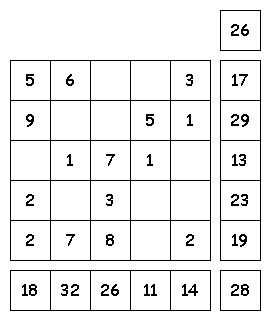Start with the rows and columns that already have four numbers.
This means the bottom row reads 2, 7, 8, 0, 2 which when added together makes 19.
So, to work out the missing number in the first column (on the left) you need to calculate 18 - (5 + 9 + 2 + 2)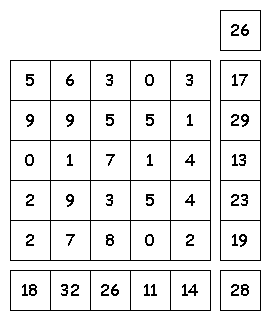Excel version of the completed 'Magic Square'

## 5: FactorisationHigher GCSE/A-Level

Find the values of x for each of the below

1. 8x2 - x = 0

2. 9x2 - 49 = 0

3. x2 - 9x + 20 = 0

4. x2 + 4x - 12 = 0

5. 8x2 + 15 = 22x

6. 20x2 = 9 + 31x

Work-through Examples:

Find the values of x when 3x2 - 2x = 0

Step 1: Factorise

x(3x - 2) = 0

Step 2: Solve

x = 0 or x = 2/3

Note: This method only works for the format ax2 + bx = 0. Otherwise, see below.

Find the values of x when 6x2 - 5x - 4 = 0

Step 1: Factorise

(2x + 1)(3x - 4)

Step 2: Solve

Either 2x + 1 = 0 or 3x - 4 = 0

So, x = -1/2 or x = 4/3

Note: If this didn't help, please visit the Maths is Fun website for a more in-depth tutorial.

1. 0 or 1/8

2. 7/3 or -7/3

3. 4 or 5

4. 2 or -6

5. 5/4 or 3/2

6. 9/5 or -1/4